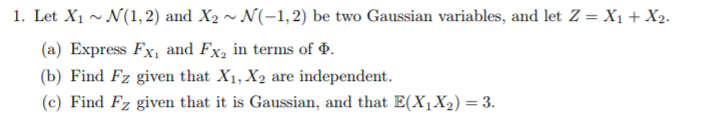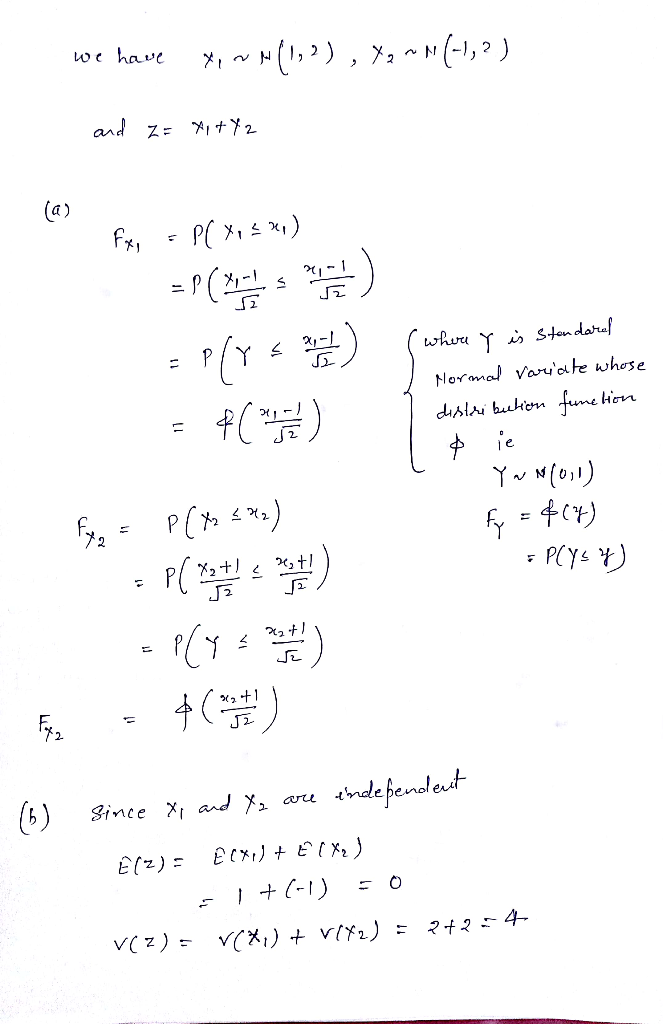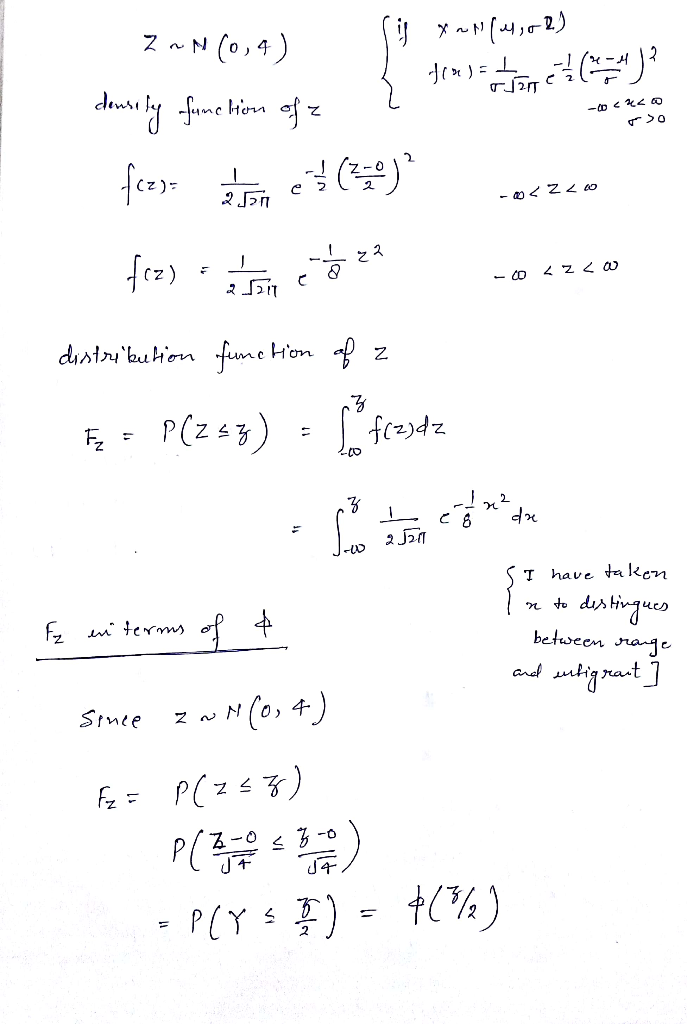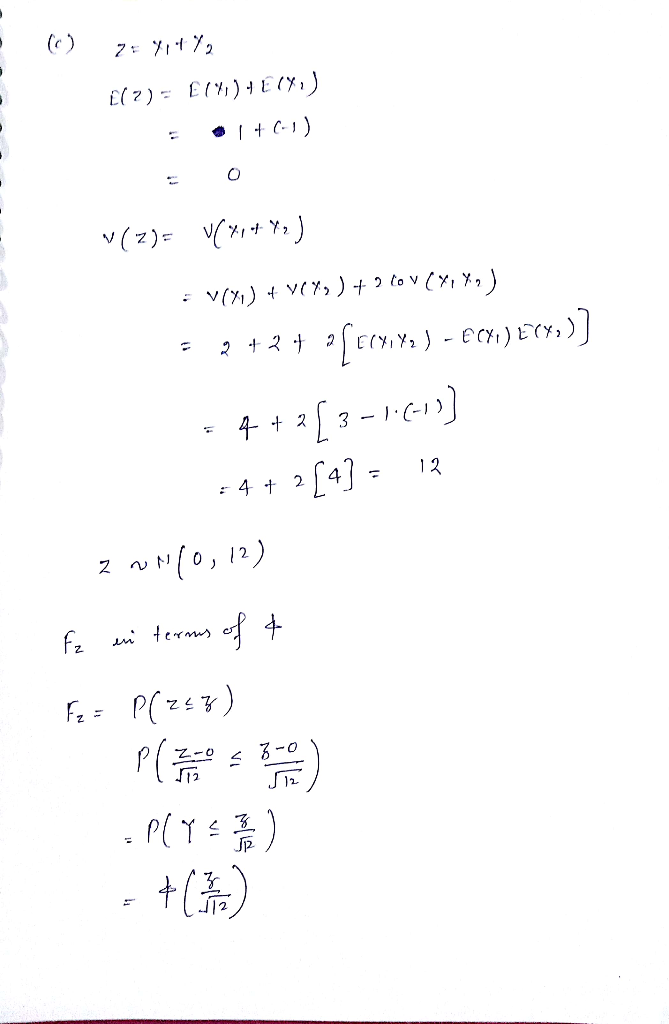Homework Help Question & Answers

1. Let X1 ~N(1,2) and X2 ~N(-1,2) be two Gaussian variables, and let Z = X1 +X2. (a) Express FX1 ...1. Let X1 ~N(1,2) and X2 ~N(-1,2) be two Gaussian variables, and let Z = X1 +X2. (a) Express FX1 and FX2 in terms of Ф. b) Find Fz given that Xi, X2 are independent. (c)Find Fz given that it is Gaussian, and that E(X2-3Add Answer of: 1. Let X1 ~N(1,2) and X2 ~N(-1,2) be two Gaussian variables, and let Z = X1 +X2. (a) Express FX1 ...
More Homework Help Questions Additional questions in this topic.

• How would I determine if a set of numbers if Gaussian or non Gaussian

Need Online Homework Help?

Get FREE EXPERT Answers
WITHIN MINUTES
Related Questions